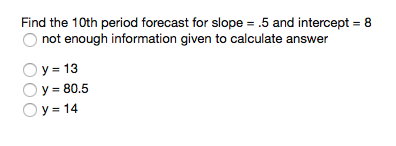# Question & Answer: Find the 10th period forecast for slope = .5 and intercept = 8 not enough information…..Find the 10th period forecast for slope = .5 and intercept = 8 not enough information given to calculate answer y = 13 y = 80.5 y = 14

The trend-projection forecasting method or linear regression method determines the value of dependent variables as follows:

Don't use plagiarized sources. Get Your Custom Essay on
Question & Answer: Find the 10th period forecast for slope = .5 and intercept = 8 not enough information…..
GET AN ESSAY WRITTEN FOR YOU FROM AS LOW AS \$13/PAGE

y = x-axis intercept + (Slope)(period number)

For the given problem. Intercept = 8, slope = 0.5 and period number = 10th period

The regression line for given problem is given as follows:

y = (8) + (0.5)(10)

y = 13

ANS: y = 13